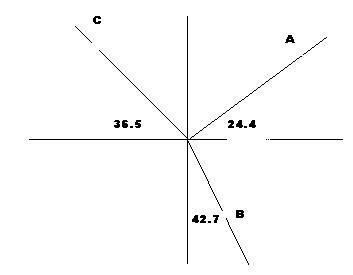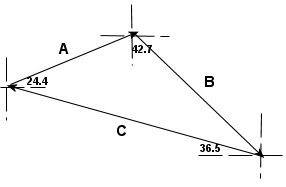# Vectors and magnitude Problem

## Homework Statement

The route followed by a hiker consists of three displacement vectors A, B and C. Vector A is along a measured trail and is 3500 m in a direction 24.4° north of east. Vector B is not along a measured trail, but the hiker uses a compass and knows that the direction is 42.7° east of south. Similarly, the direction of vector C is 36.5° north of west. The hiker ends up back where she started, so the resultant displacement is zero, or A + B + C = 0. Calculate the magnitude of vector B.

## Homework Equations

A + B + C = 0

a^2 + b^2 = c^2

a/sinA = b/sinB = c/sinC

## The Attempt at a Solution

I worked this one out completely to what I believed to be the answer. I first set the vectors up in head to tail fashion so they created a triangle, I then used the law of sines to determine angle B which I know is definitely right, so I'm thinking is that where my error lies is the angles of A and C. When I went over this recitation for some reason I had that C = 60.5 deg, but that's only part of the angle right? Shouldn't that be added to 24.4? But if I add those together A sums up to be about 10.8 which makes no sense at all so I'm definitely doing something wrong.

If I were to do the problem, I would extend vectors A, B, and C out from the origin. (It might be convenient at this point to rotate the figure by a certain amount so that a vector lines up with an axis.) We know the x and y components of A. Let b and c be the magnitudes of vectors B and C. We can get the x and y components of vectors B and C, and making sure all the components along each axis add to zero, we can get two equations with the two unknowns b and c, which we can then solve for.

So you're taking the approach of just drawing each vector out on a coordinate system? I tried to use that method, but it only confused me, the triangle way was easier for me to follow. So if I do it that way should it look like this or are my angles in the wrong place?sorry the graph looks so shotty, I quickly did it in paint.

D H
Staff Emeritus
The triangle approach will work just fine here. Two questions:
• Why did you use the law of sines to compute the angle B when the angles are completely determined from the problem statement? The law of sines is the right thing to use, but you should use it to compute the length of vector B, not angle B.
• What do you mean by the angle B? One obvious naming scheme is to name the angles by the vector opposite the angle.

The triangle approach will work just fine here. Two questions:
• Why did you use the law of sines to compute the angle B when the angles are completely determined from the problem statement? The law of sines is the right thing to use, but you should use it to compute the length of vector B, not angle B.
• What do you mean by the angle B? One obvious naming scheme is to name the angles by the vector opposite the angle.

the law of sines approach was for the triangle approach. Given that approach, when you line the vector up head to tail the angles given are not the angles within the triangle. I'm gonna draw it up for you real quick, forgive me if it looks really bad.the dashed lines signify the axis the vector is being added to relative to the tip to tail method. As you can see the angles given aren't the actual angles of the triangle. And when I said angle B I was in fact referring to the angle opposite vector B.

D H
Staff Emeritus
The diagram is fine. Now why do you need the law of sines to compute angle B? Angle B is 24.4 degrees plus some other angle. Hint: That other angle corresponds to an angle on your diagram.

angle is equal to 24.4 + 36.5 = 60.9 degrees, my bad you wouldn't use the law of sines to find angle B but rather to find side B. My real problem is the other angles. I know you can find angle C by drawing a right triangle with side A as your hypotenuse but then when you find that number and add it 42.7, the sum of that angle plus B would have A being 10.8 which isn't right, so I must be doing something wrong.

Last edited:
D H
Staff Emeritus
10.8 degrees is the right value for angle A. What makes you think it is wrong?

As a check, on your work, compute the lengths of vectors B and C via the law of sines. Compute the locations of the ends of vector A, vector A+B, and vector A+B+C. The latter should be right back at the origin.

So you're taking the approach of just drawing each vector out on a coordinate system? I tried to use that method, but it only confused me, the triangle way was easier for me to follow. So if I do it that way should it look like this or are my angles in the wrong place?sorry the graph looks so shotty, I quickly did it in paint.

That's the graph I was thinking of.

you know what? I just worked the problem out now and got an answer, checked it with the actual answer and it's right. I feel really stupid now, because I was racking my brain trying to get the right answer when I was doing this problem two weeks ago and I do it now and it's right, despite the fact that I could have sworn I followed the same exact procedure when I did then. Well, thanks for the help I guess I wasn't doing it wrong.